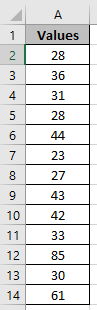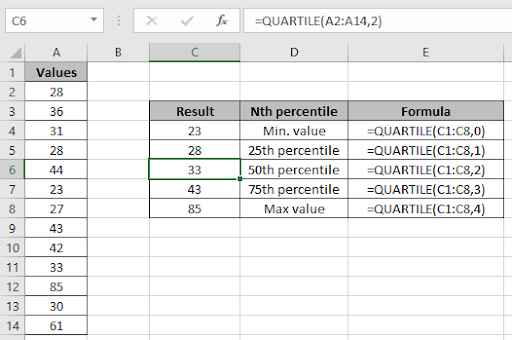# How to use the Excel Quartile function

Quartile function returns the nth percentile of the range of values.
It returns minimum value, 1/4th quartile value, 1/2th quartile value, 3/4th value & maximum value using the quart argument.
Syntax:

=QUARTILE(array, quart)
 Quart Nth percentile 0 0th percentile or Min Value 1 25th percentile 2 50th percentile 3 75th percentile 4 100th percentile or Max Value

Let’s run some tests on some numbers using ABS function
The nth percentile value for the range of values : 0,1,2,3,4
Use the formula:

=QUARTILE(H2:H6,0)

The red cells are the negative numbers provided in different formatting styles.

Now we wish to find the quartile of the data setUse the formula

=QUARTILE(A2:A14,0)As you can see the quartile of the range using Excel Quartile function.

Hope you understood Excel Quartile function. Explore more articles on mathematical Excel functions here. Please state your queries in the comment box below.

Popular Articles:

50 Excel Shortcuts to Increase Your Productivity

How to use the VLOOKUP Function in Excel

How to use the COUNTIF function in Excel 2016

How to Use SUMIF Function in Excel

Terms and Conditions of use

The applications/code on this site are distributed as is and without warranties or liability. In no event shall the owner of the copyrights, or the authors of the applications/code be liable for any loss of profit, any problems or any damage resulting from the use or evaluation of the applications/code.